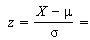# Normal Probability Practice Problems and Answers

Share on

Probability and Statistics > Probability > Normal Probability Practice Problems

These normal probability practice problems will help you practice calculating z-scores and using the z-table.General steps for solving normal probability practice problems:

1. Use the equation above to find a z-score. If you don’t know how to look up z-scores (or if you want more practice, see this z-score article for videos and step-by-step instructions.
2. Look up the z-score in the z-table and find the area.
3. Convert the area to a percentage.

If you more need help with these steps, see the normal distribution word problems index, which outlines the steps in detail (with videos).

## Normal Probability Practice Problems.

Click on the question for the answer!

1. Scores on a particular test are normally distributed with a standard deviation of 4 and a mean of 30. What is the probability of anyone scoring less than 40?

2. Annual salaries for a large company are approximately normally distributed with a mean of \$50,000 and a of \$20,000. What percentage of company workers may under \$40,000?

4. The amount of time a student taking statistics spends on studying for a test is normally distributed. If the average time spent studying is 12 hours and the standard deviation is 4 hours, what is the probability that a student will spend more than 8 hours studying?

5. The amount of candy dispensed by a candy machine is normally distributed with a mean of 0.9 oz and a standard deviation of 0.1 ounces. If the machine is used 500 times, how many times will it dispense more than 1 oz of candy?

Check out our YouTube channel for more stats help and tips!

CITE THIS AS:
Stephanie Glen. "Normal Probability Practice Problems and Answers" From StatisticsHowTo.com: Elementary Statistics for the rest of us! https://www.statisticshowto.com/normal-probability-practice-problems/
---------------------------------------------------------------------------Need help with a homework or test question? With Chegg Study, you can get step-by-step solutions to your questions from an expert in the field. Your first 30 minutes with a Chegg tutor is free!